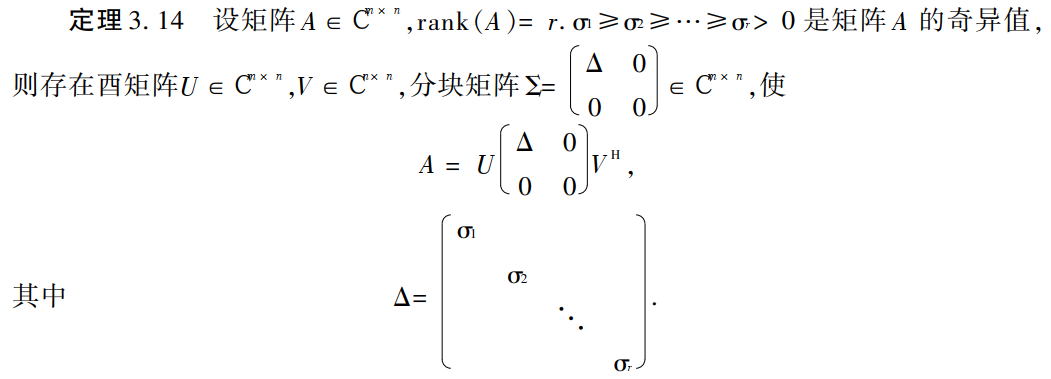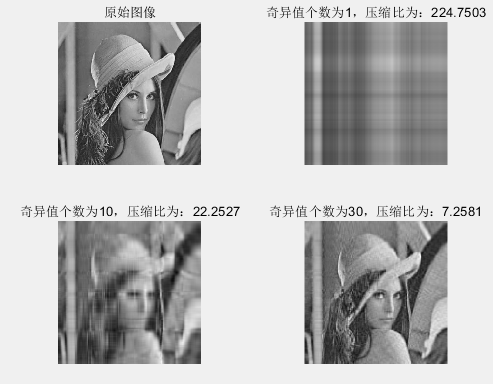# 矩阵的奇异值分解及其在图像压缩领域的作用

4 篇文章 1 订阅

1.矩阵奇异值分解定义及数学原理

矩阵的奇异值分解英文全称为Singular value decomposition，简称SVD分解。

基本数学原理如下：那么矩阵的奇异值分解是怎样实现图像压缩的呢？假设一副为height*width的图像，则原图的像素点个数为height*width个，进行奇异值为r的分解之后，像素点就变为height*r+r*r+width*r个，压缩比为（height*width）/（height*r+r*r+width*r），使得存储空间大大降低。

2.奇异值分解的MATLAB程序简单仿真

我们在MATLAB中通过调用svd这个内置函数，来简单看一下矩阵奇异值分解的一个具体实际效果，很简单的一段代码，代码如下：

A=imread('timg.jpg');%读入图像
A=rgb2gray(A);%rgb转灰度
[m,n]=size(A);
subplot(2,2,1);
imshow(A);
title('原始图像');
[U,S,V]=svd(double(A),'econ');%进行奇异值分解
r1=1;%奇异值个数
B=U(:,1:r1)*S(1:r1,1:r1)*V(:,1:r1)';
B=uint8(B);
subplot(2,2,2);
imshow(B);
ysb1=(m.*n)./(m.*r1+r1.*r1+n.*r1);
title(['奇异值个数为1，压缩比为：',num2str(ysb1)]);
r2=10;%奇异值个数
C=U(:,1:r2)*S(1:r2,1:r2)*V(:,1:r2)';
C=uint8(C);
subplot(2,2,3);
imshow(C);
ysb2=(m.*n)./(m.*r2+r2.*r2+n.*r2);
title(['奇异值个数为10，压缩比为：',num2str(ysb2)]);
r3=30;%奇异值个数
D=U(:,1:r3)*S(1:r3,1:r3)*V(:,1:r3)';
D=uint8(D);
subplot(2,2,4);
imshow(D);
ysb3=(m.*n)./(m.*r3+r3.*r3+n.*r3);
title(['奇异值个数为30，压缩比为：',num2str(ysb3)]);

结果如图所示 ：从图中可以看出，当奇异值为1时，图像完全模糊，看不清楚，当奇异值为10时，图像初显轮廓，可以看的比较清楚，当奇异值为30的时候，图像已经很清楚了，此时的压缩比7.2581，即像素点的个数相比原图减少了7.2581倍，从而存储空间得到大大降低。

03-24
05-251万+04-241666
12-081078
12-246367
01-163636
04-239329
01-176203
03-283966
06-203万+
11-22
04-222392
09-25426
07-082304
06-015007
07-1612万+
07-116471
07-116015点击重新获取扫码支付余额充值